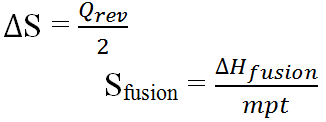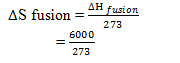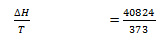# Entropy Function

Entropy function was introduced by Clausius. Entropy of a system is the measurement of disorderness of randomness or chausness of the system.
S = k ln w, where
K = Bollzmann’s constant
ln = loge
w = no of probable arrangements

Entropy is a state function and depends only upon initial and final states, also change in entropy ( s) will be
Δ S = S2 – S1
Where S2 = Final state entropy
S1 = Entropy of Initial state
In chemical reaction, the change in entropy is,
Δ Sreaction = Sproducts – Sreactants

In solid, the molecules are arranged orderly so it has the least entropy but in liquid the disorderness is more than solid while in gas, the disoderness is maximum. So the order of entropy of a substance in different physical states, is,
Ssolid < Sliquid << Sgas

The change in entropy during a physical transition at transition temperature is ratio of heat absorbed or released to transition temperature. i.e.Its unit is Jmol-1K-1

The enthalpy of fusion of water is 6KJmole-1. Calculate the entropy of fusion of water at its melting point.
Sol: Given
ΔH fusion = 6KJmole-1 = 6000 Jmole-1
Mpt of water (T) = 00c = 273 K
ΔS fusion = ?
We have,=21.98 Jmole-1K-1

The latent heat of vaporization of water is 2268Jg-1. Calculate the molar entropy of vaporization of water at its normal boiling point.
ΔH vaporization (Enthalpy of Vaporization) = 2268 Jg-1
= 2268 × 18 = 40824 Jmol-1
Bpt of water (T) = 373 K
Then,
Entropy of vaporization, ( ΔS) == 109.44 J.mol-1k-1